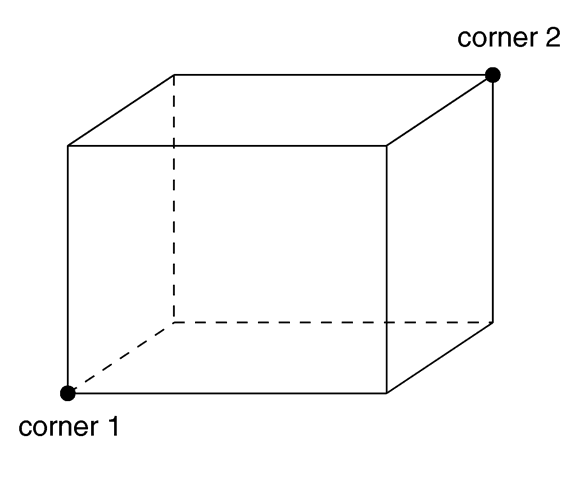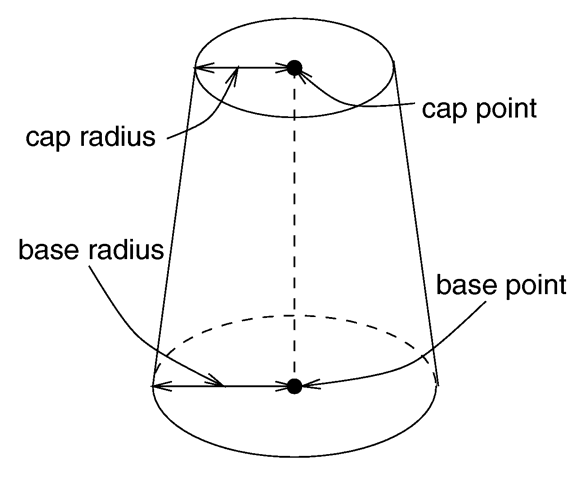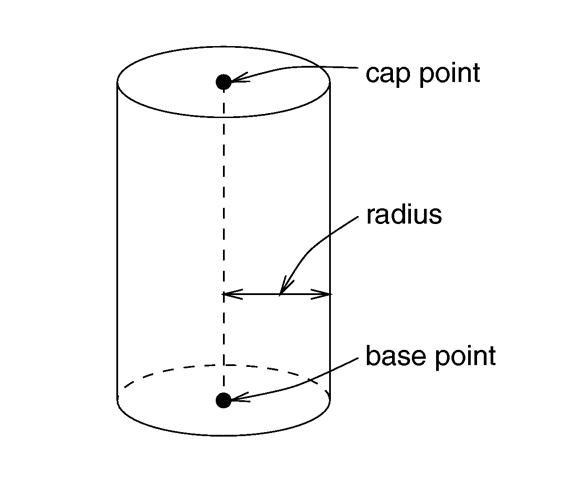### Section 7.5.2Finite Solid Primitives

There are twelve different solid finite primitive shapes: blob, box, cone, cylinder, fractal, height field, lathe, sphere, superellipsoid, surface of revolution, text object and torus. These have a well-defined inside and can be used in CSG (see section "Constructive Solid Geometry"). They are finite and respond to automatic bounding. As with all shapes they can be translated, rotated and scaled.

### Section 7.5.2.1Blob

Blobs are an interesting and flexible object type. Mathematically they are iso-surfaces of scalar fields, i. e. their surface is defined by the strength of the field in each point. If this strength is equal to a threshold value you're on the surface otherwise you're not.

Picture each blob component as an object floating in space. This object is filled with a field that has its maximum at the center of the object and drops off to zero at the object's surface. The field strength of all those components are added together to form the field of the blob. Now POV-Ray looks for points where this field has a given value, the threshold value. All these points form the surface of the blob object. Points with a greater field value than the threshold value are considered to be inside while points with a smaller field value are outside.

There's another, simpler way of looking at blobs. They can be seen as a union of flexible components that attract or repel each other to form a blobby organic looking shape. The components' surfaces actually stretch out smoothly and connect as if they were made of honey or something like that.

blob { threshold THRESHOLD_VALUE cylinder { <END1>, <END2>, RADIUS, [ strength ] STRENGTH } sphere { <CENTER>, RADIUS, [ strength ] STRENGTH } [ component STRENGTH, RADIUS, <CENTER> ] [ hierarchy FLAG ] [ sturm ] }

 1) The threshold value must be positive. 2) A component disappears if the threshold value is greater than its strength. 3) As the threshold value gets larger the surface you see gets closer to the centers of the components. 4) As the threshold value gets smaller, the surface you see gets closer to the surface of the components.

sphere { <CENTER>, RADIUS, [strength] STRENGTH [ translate <VECTOR> ] [ rotate <VECTOR> ] [ scale <VECTOR> ] TEXTURE_MODIFIERS } cylinder { <END1>, <END2>, RADIUS, [strength] STRENGTH [ translate <VECTOR> ] [ rotate <VECTOR> ] [ scale <VECTOR> ] TEXTURE_MODIFIERS }

You should keep the following things in mind.

 1) The strength value may be positive or negative. Zero is a bad value, as the net result is that no field was added --- you might just as well have not used this component. 2) If strength is positive, then POV-Ray will add the component's field to the space around the center of the component. If this adds enough field strength to be greater than the "threshold" value you will see a surface. 3) If the strength value is negative, then POV-Ray will subtract the component's field from the space around the center of the component. This will only do something if there happen to be positive components nearby. What happens is that the surface around any nearby positive components will be dented away from the center of the negative component.

An example of a three component blob is:

blob { threshold 0.6 sphere { <.75, 0, 0>, 1, 1 } sphere { <-.375, .64952, 0>, 1, 1 } sphere { <-.375, -.64952, 0>, 1, 1 } scale 2 }

If you have a single blob component then the surface you see will just look like the object used, i. e. a sphere or a cylinder, with the surface being somewhere inside the surface specified for the component. The exact surface location can be determined from the blob equation listed below (you will probably never need to know this, blobs are more for visual appeal than for exact modeling).

For the more mathematically minded, here's the formula used internally by POV-Ray to create blobs. You don't need to understand this to use blobs. The formula used for a single blob component is:

density = strength * (1 - radius^2)^2

This formula has the nice property that it is exactly equal to the strength parameter at the center of the component and drops off to exactly 0 at a distance from the center of the component that is equal to the radius value. The density formula for more than one blob component is just the sum of the individual component densities:

density = density1 + density2 + ...

### Section 7.5.2.2Box

A simple box can be defined by listing two corners of the box like this:

box { <CORNER1>, <CORNER2> }The geometry of a box.

Where <CORNER1> and <CORNER2> are vectors defining the x, y, z coordinates of the opposite corners of the box.

Each element of <CORNER1> should always be less than the corresponding element in <CORNER2>. If any elements of <CORNER1> are larger than <CORNER2> the box will not appear in the scene.

Boxes are calculated efficiently and make good bounding shapes (if manually bounding seems to be necessary).

### Section 7.5.2.3Cone

A finite length cone or a frustum (a cone with the point cut off) may be defined by.The geometry of a cone.

### Section 7.5.2.4Cylinder

A finite length cylinder with parallel end caps may be defined by.

cylinder { <BASE_POINT>, <CAP_POINT>, RADIUS [ open ] }The geometry of a cylinder.

Next Section